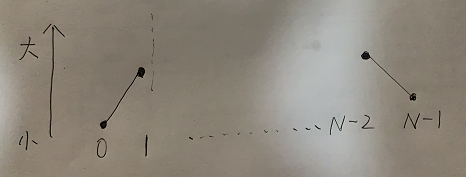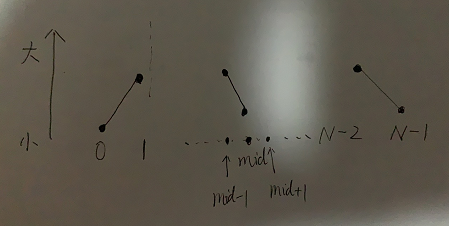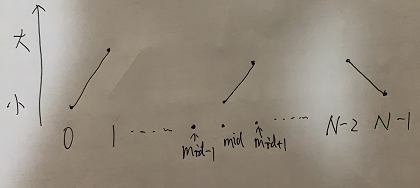# 使用二分法來解決的問題

作者：Grey

原文地址：使用二分法來解決的問題

## 在一個有序數組中，找某個數是否存在

思路：

1. 先得到中點位置，中點可以把數組分為左右半邊。
2. 如果中點位置的值等于目標值，直接返回中點位置。
3. 如果中點位置的值小于目標值，則去數組左邊按同樣的方式尋找。
4. 如果中點位置的值大于目標值，則取數組右邊按同樣的方式尋找。
5. 如果最后沒有找到，則返回：-1。

代碼

class Solution {
public int search(int[] nums, int target) {
if(nums == null || nums.length < 1) {
return -1;
}
int l = 0;
int r = nums.length - 1;
while (l <= r) {
int mid = l + ((r - l)>>1);
if (target > nums[mid]) {
l = mid + 1;
} else if (target == nums[mid]) {
return mid;
} else {
r = mid - 1;
}
}
return -1;
}
}


## 在一個有序數組中，找大于等于某個數最左側的位置

OJ見：?？?查找某個位置

這個問題只需要在上例基礎上進行簡單改動即可，上例中，我們找到滿足條件的位置就直接return

if (target == nums[mid]) {
return mid;
}


在本問題中，因為要找到最左側的位置，所以，在遇到相等的時候，只需要先把位置記錄下來，不用直接返回，然后繼續去左側找是否還有滿足條件的位置。

同時，在遇到target < nums[mid]條件下，也需要記錄下此時的mid位置，因為這也可能是滿足條件的位置。

代碼：

import java.util.Arrays;
import java.util.Scanner;

public class Main {
public static void main(String[] args) {
Scanner in = new Scanner(System.in);
int n = in.nextInt();
int k = in.nextInt();
int[] arr = new int[n];
for (int i = 0; i < n; i++) {
arr[i] = in.nextInt();
}
// 雖然不排序也可以通過，但是題目未說明一定是有序數組
Arrays.sort(arr);
System.out.println(getNearestLeft(arr, k));
in.close();
}

public static int getNearestLeft(int[] nums, int target) {
if (nums == null || nums.length < 1) {
return -1;
}
int l = 0;
int r = nums.length - 1;
int ans = 0;
while (l <= r) {
int mid = l + ((r - l) >> 1);
if (target < nums[mid]) {
ans = mid;
r = mid - 1;
} else if (target > nums[mid]) {
l = mid + 1;
} else {
ans = mid;
r = mid - 1;
}
}
return ans;
}
}


LeetCode上有很多類似的問題，都可以用如上方式解答，比如：

LeetCode 35. Search Insert Position

LeetCode 34. Find First and Last Position of Element in Sorted Array

## 局部最大值問題

思路

假設數組長度為N，首先判斷0號位置的數和N-1位置的數是不是峰值位置。

0號位置只需要和1號位置比較，如果0號位置大，0號位置就是峰值位置。

N-1號位置只需要和N-2號位置比較，如果N-1號位置大，N-1號位置就是峰值位置。

如果0號位置和N-1在上輪比較中均是最小值，那么數組的樣子必然是如下情況：[0..1]區間內是增長, [N-2...N-1]區間內是下降

那么峰值位置必在[1...N-2]之間出現

此時可以通過二分來找峰值位置，先來到中點位置，假設為mid，如果:

arr[mid] > arr[mid+1] && arr[mid] > arr[mid-1]


mid位置即峰值位置

否則，有如下兩種情況：

情況一，趨勢是：則在[1...(mid-1)]區間內繼續上述二分。

情況二，趨勢是：則在[(mid+1)...(N-2)]區間內繼續上述二分。

完整代碼

public class LeetCode_0162_FindPeakElement {
public static int findPeakElement(int[] nums) {
if (nums.length == 1) {
return 0;
}
int l = 0;
int r = nums.length - 1;
if (nums[l] > nums[l + 1]) {
return l;
}
if (nums[r] > nums[r - 1]) {
return r;
}
l = l + 1;
r = r - 1;
while (l <= r) {
int mid = l + ((r - l) >> 1);
if (nums[mid] > nums[mid + 1] && nums[mid] > nums[mid - 1]) {
return mid;
}
if (nums[mid] < nums[mid + 1]) {
l = mid + 1;
} else if (nums[mid] < nums[mid - 1]) {
r = mid - 1;
}
}
return -1;
}
}



## 分割數組的最大值

給定一個非負整數數組 nums 和一個整數 m ，你需要將這個數組分成 m 個非空的連續子數組。設計一個算法使得這 m 個子數組各自和的最大值最小。

PS: 此題也可以用四邊形不等式優化的動態規劃來解，但是最優解是二分法

思路

我們先求整個數組的累加和，假設累加和為sum，我們可以得到一個結論，分割的m個非空連續子數組的和的范圍一定在(0,sum]區間內。轉換一下思路，如果某種劃分下的子數組之和的最大值為max，則max首先肯定在(0,sum]區間內。思路轉換為：

子數組的累加和最大值不能超過max的情況下，最少可分多少部分？

假設能分k個部分，

如果k <= m，說明這種劃分是滿足條件的，我們看max是否可以變的更小。

如果k > m，說明這種劃分是不滿足條件的，我們需要調大max的值。

這里可以通過二分的方式來定位max的值。即max先取(0,sum]的中點位置，得到的劃分部分k如果k <= m，則max繼續去左邊取中點位置來得到新的劃分k，

如果k > m，max繼續從右邊的中點位置來得到新的劃分k。

完整代碼

class Solution {
public static int splitArray(int[] nums, int m) {
int sum = 0;
for (int num : nums) {
sum += num;
}
int l = 0;
int r = sum;
int ans = 0;
while (l <= r) {
int mid = l + ((r - l) >> 1);
int parts = getParts(nums, mid);
if (parts > m) {
// mid越大，parts才會越小
l = mid + 1;
} else {
ans = mid;
r = mid - 1;
}
}
return ans;
}

// 達到aim要分幾部分
public static int getParts(int[] nums, int aim) {
for (int num : nums) {
if (num > aim) {
return Integer.MAX_VALUE;
}
}
int part = 1;
int all = nums;
for (int i = 1; i < nums.length; i++) {
if (all + nums[i] > aim) {
part++;
all = nums[i];
} else {
all += nums[i];
}
}
return part;
}
}


其中：int getParts(int[] nums, int aim)方法表示，在不超過aim的情況下，最少需要幾個劃分部分。方法的主要邏輯是：

遍歷數組，如果發現某個元素的值超過了aim，直接返回系統最大，說明無法得到劃分。如果沒有超過aim，則繼續加入下一個元素，直到超過aim，就定位出一個部分。依次類推，就可以得到最少有幾個劃分。

算法和數據結構筆記

## 參考資料

算法和數據結構體系班-左程云

posted @ 2022-03-07 19:05  Grey Zeng  閱讀(115)  評論(0編輯  收藏  舉報
国产在线码观看超清无码视频,人妻精品动漫H无码,十大看黄台高清视频,国产在线无码视频一区二区三区,国产男女乱婬真视频免费,免费看女人的隐私超爽,狠狠色狠狠色综合久久蜜芽